# Transition Elements

## Transition Element General Description

There are two Flow Simulator transition elements available. Both can be used in compressible flow and incompressible flow simulations. This element is used to model various types of expansions (Ex: Flow through diffuser, Flow through an abrupt expansion) and contractions (Ex: Flow through reducers, Flow through a sudden contraction). These include abrupt and conical shaped as well as parameter inputs for defining the behavior of arbitrary shaped expansions and contractions.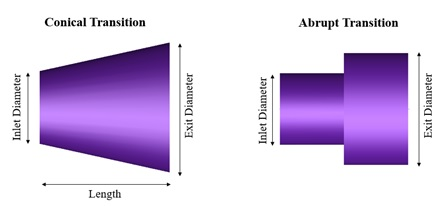## Quick Guide for Transition Element Creation in the GUI

Both Conical & Abrupt Transitions can be found under both “Compressible Gas Elements” & “Incompressible Liquid Elements” - “Expansions & Contraction” section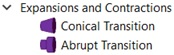The geometric inputs like inlet diameter, exit diameter and length are mandatory inputs. Apart from these, inputs related to friction are required if friction options are selected. Descriptions about each input is described below in “Transition Element Inputs” section.

Note: The GUI automatically flips the element image on the flow network model based on user inputs for inlet & exit diameter to correctly indicate the expansions or contraction type geometry.

## Transition Element Inputs

Table of the inputs specific for the Conical Transition Element.

 Conical Transition Element Input Variables Index UI Name (. flo label) Description 1 Expansion Type (EXPAN_TYPE) Type of expansion. 10:Expansion with Fixed K Loss. 30: Conical Expansion – Blevins 31: Conical Expansion – Miller 40: Expansion with Fixed Cp (Static Pressure Recovery Coefficient) 50: Expansion with Fixed Effectiveness 60: CD Loss 2 Contraction Type (CONTRAC_TYPE) Type of contraction. 70:Contraction with Fixed K Loss. 90: Conical Contraction – Blevins 91: Conical Contraction - Miller 3 Expansion Parameter (K_EXPAN) If EXPAN_TYPE=10, then K_EXPAN is an expansion K loss (Total Pressure Loss Coefficient). If EXPAN_TYPE=40, then K_EXPAN is a diffuser Cp If EXPAN_TYPE=50, then K_EXPAN is a diffuser Effectiveness If EXPAN_TYPE=60, then K_EXPAN is the CD Loss Parameter. For other expansion types, the expansion parameter is determined by equations or table lookups, so this input is not required. 4 Contraction Parameter (K_CONTRAC) If CONTRAC_TYPE=70, then K_CONTRAC is a contraction K loss For other contraction types, the contraction parameter is determined by equation or table lookups, so this input is not required. 5 Length (LENGTH) Length is used for calculation of the expansion/contraction angle of the conical types and is used in the additional friction calculation. 6 Turbulent Friction Relation (FRIC_MODE) Friction options 0: Friction Off 1: Friction calculated with Swamee-Jain approximation to Colebrook-White (Moody Diagram) 2: Friction specified by user in the FRICTION field. 7 Darcy Friction Coefficient (FRICTION) When FRIC_MODE=2 (User-specified friction), Darcy Friction Coefficient should be provided. 8 Roughness (ROUGHNESS) Roughness value When FRIC_MODE=1 (friction calculated with Swamee-Jain approximation to Colebrook-White), roughness can be provided. Sand-grain roughness is the default type, since that is what Moody, Colebrook-White, Swamee, etc. are based on. 9 Friction Multiplier (FMULT) A user-specified multiplier for friction. This should only be used with calculated friction values. FMULTs are often used to account for roughness effects, so FMULT should probably be used only when ROUGHNESS =0. 10 Roughness Type (ROUGH_TYPE) This tells what type of rough the ROUGHNESS value is. 0: Sand-Grain Roughness 1: Average-Absolute Roughness 2: Root-Mean-Square Roughness 3: Peak-to-Valley Roughness

Table of the inputs specific for the Abrupt Transition Element.

 Abrupt Transition Element Input Variables Index UI Name (. flo label) Description 1 Expansion Type (EXPAN_TYPE) Type of expansion. 10:Expansion with Fixed K Loss. 20: Abrupt Expansion – Simple 22: Abrupt Expansion – Miller 2 Contraction Type (CONTRAC_TYPE) Type of contraction. 70:Contraction with Fixed K Loss. 80: Abrupt Contraction – Simple 81: Abrupt Contraction – Polynomial 82: Abrupt Contraction – Miller1 83: Abrupt Contraction – Miller2 3 Expansion Parameter (K_EXPAN) If EXPAN_TYPE=10, then K_EXPAN is an expansion K loss (Total Pressure Loss Coefficient). For other expansion types, the expansion parameter is determined by equations or table lookups, so this input is not required. 4 Contraction Parameter (K_CONTRAC) If CONTRAC_TYPE=70, then K_CONTRAC is a contraction K loss For other contraction types, the contraction parameter is determined by equation or table lookups, so this input is not required. 5 Minor Fillet Radius (FRAD_MINOR) Fillet Radius at the smaller diameter location

Table of the common inputs for both Conical & Abrupt Transition Element.

Transition Element Common Input Variables
Index UI Name (.flo label) Description
1 Cross-Section Shape (CS_MODE) Cross-section shape allows user to specify geometry in three different ways.

1: Circular with Inlet and Exit Area specified (Internally, the solver computes diameters and perimeters.)

2: Circular with Inlet and Exit Diameter specified (Internally, the solver computes areas and perimeters)

3: Arbitrary Shape with Inlet and Exit Area as well as Inlet and Exit Hydraulic Diameter specified. (Internally, the solver computes the perimeters.)

2 Inlet Area (INLET_AREA) Area at transition inlet

If INLET_AREA is greater than EXIT_AREA, then the transition is understood to be a contraction when flow is in the forward direction (from the user-defined upstream chamber to the user-defined downstream chamber). If the flow direction is reversed in the final solution, the transition element treats the channel as an expansion (using the EXPAN_TYPE input, etc.).

3 Exit Area (EXIT_AREA) Area at transition exit

If EXIT_AREA is greater than INLET_AREA, then the transition is understood to be an expansion when flow is in the forward direction (from the user-defined upstream chamber to the user-defined downstream chamber). If the flow direction is reversed in the final solution, the transition element treats the channel as a contraction (using the CONTRAC_TYPE input, etc.).

4 Inlet Hydraulic Diameter (INLET_DH) Diameter (or Hydraulic Diameter) at transition inlet

Same flow direction logic as explained in the INLET_AREA section

5 Exit Hydraulic Diameter (EXIT_DH) Diameter (or Hydraulic Diameter) at transition exit

Same flow direction logic as explained in the EXIT_AREA section

7 Element Inlet Orientation: Tangential Angle (THETA) Angle between the element centerline at the entrance of the element and the reference direction.

If the element is rotating or directly connected to one or more rotating elements, the reference direction is defined as parallel to the engine centerline and the angle is the projected angle in the tangential direction. Otherwise, the reference direction is arbitrary but assumed to be the same as the reference direction for all other elements attached to the upstream chamber.

THETA for an element downstream of a plenum chamber has no impact on the solution except to set the default value of THETA_EX.

8 Element Inlet Orientation: Radial Angle (PHI) Angle between the element centerline at the entrance of the element and the THETA direction. (spherical coordinate system)

PHI for an element downstream of a plenum chamber has no impact on the solution except to set the default value of PHI_EX.

9 Exit K Loss:

Axial (K_EXIT_Z)

Tangential (K_EXIT_U)

Head loss factors in the Z, U, and R directions based on the spherical coordinate system of theta and phi. (Default value provides no loss).

10 Element Exit Orientation: Tangential Angle (THETA_EX) Angle between the orifice exit centerline and the reference direction.

THETA_EX is an optional variable to be used if the orientation of the element exit differs from that of the element inlet.

The default value (THETA_EX = -999) will result in the assumption that THETA_EX = THETA.

Other values will be interpreted in the manner presented in the description of THETA.

11 Element Exit Orientation: Radial Angle (PHI_EX)

Angle between the orifice exit centerline and the THETA_EX direction.

PHI_EX is an optional variable to be used if the orientation of the element exit differs from that of the element inlet.

The default (PHI_EX = -999) will result in the assumption that PHI_EX = PHI.

Other values will be interpreted in the manner presented in the description of PHI.

12 Heat Addition Mode (HEAT_MODE) Mode of heat transfer to/from the fluid in the element.

13 Heat Added (QIN) The value entered for QIN depends on the HEAT_MODE chosen.

14 Fluid Compressibility Mode (FLUID_MODE)

The user can choose which solution algorithm to use.

1: Compressible Fluid

2: Incompressible Fluid

3: Compressible with Compressible K

4: Incompressible liquid

15 Reynolds Number Correction Relation for K

(RE_CORR)

The user can choose which to adjust K (or Cp) based according to the following options.

0: Off

1: General Purpose – Recommended for abrupt expansion, abrupt contraction, and valves

2: Diffuser correction & Conical Expansion – Recommended for diffusers

## Transition Element Theory

The transition element module applies conservation of mass, momentum, and energy equations to relate static pressure, total pressure, total temperature, and flow rate at the element inlet with those at the element exit. All contractions are governed by contraction Kloss for their momentum equation. Expansion elements are divided into two categories: Those that use expansion K and those that use diffuser CP for the momentum equation. All transitions break down into these three different momentum equation types. In addition, transitions use one of three different gas assumptions:

1. Incompressible relation between Pt and Ps, and incompressible K definition
2. Compressible relation between Pt and Ps, and incompressible K definition. (Recommended for general usage)
3. Compressible relation between Pt and Ps, and compressible K definition. (Recommended only if K is defined compressible)

Expansion – Kloss Calculations

Expansion K is calculated differently based on the “Expansion Type” selected for the transition element. The K formulations for the different expansion types are outlined below.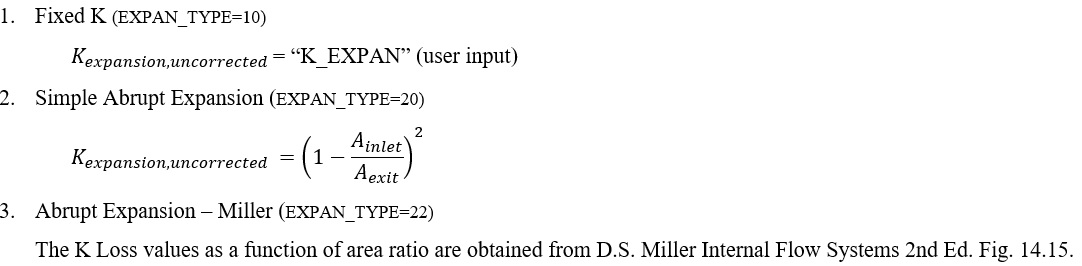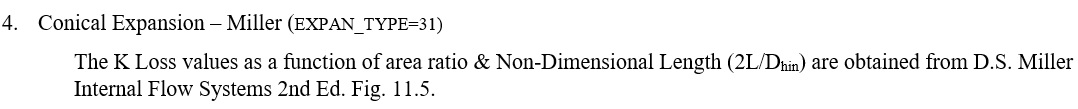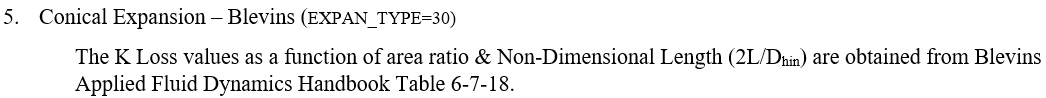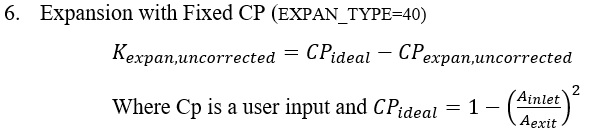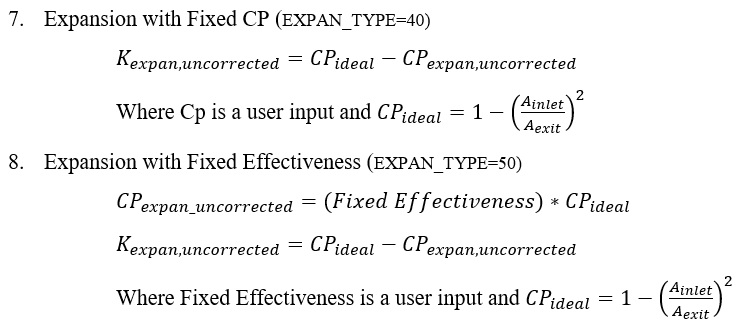Contraction – Kloss Calculations

Contraction K is calculated differently based on the “Contraction Type” selected for the transition element. The K formulations for the different expansion types are outlined below.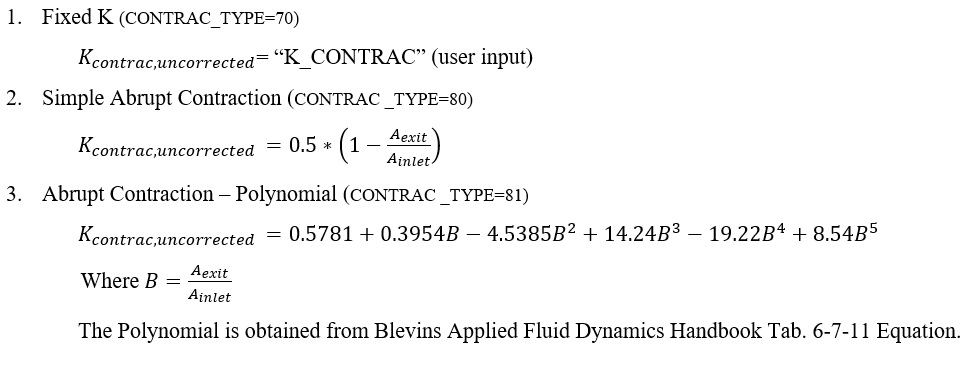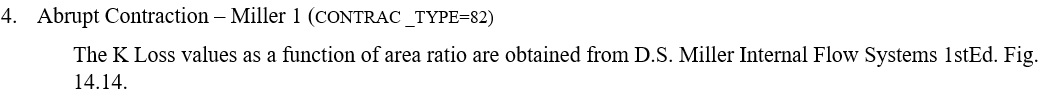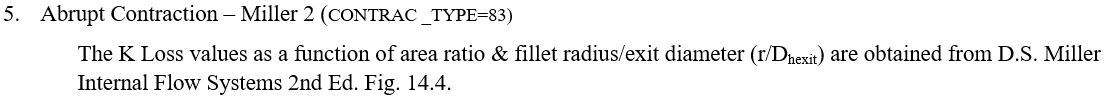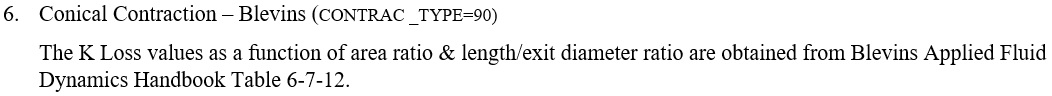Note: Flow Simulator uses linear interpolation to obtain values from the curves, & it doesn’t do any extrapolation if the values are outside the prescribed input limits. A detailed error & warning messages are written out in Convhist_fi.out file. The below table summarizes the error & warning limit for each expansion & contraction coefficient options.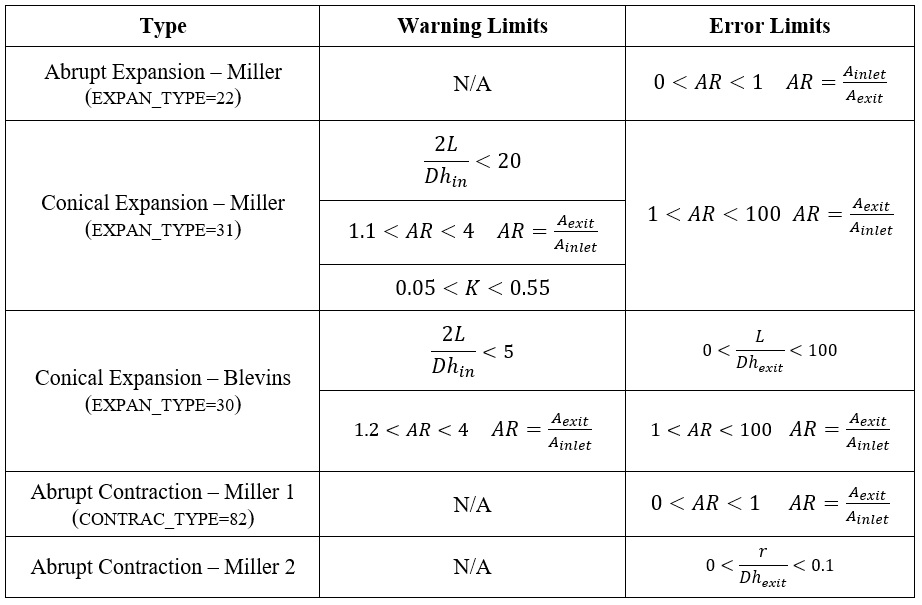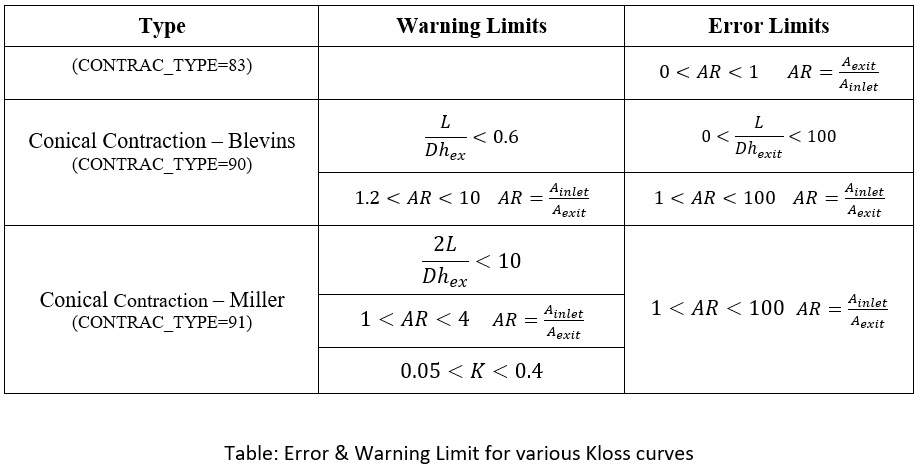Kloss (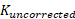) as calculated using the above formulations will be corrected if opted to account for Reynolds numbers using one of the options mentioned below. The details of correction factors are available under General solver theory sections

1. General Purpose – Recommended for abrupt expansion, abrupt contraction, and valves (RE_CORR = 1)
2. Diffuser correction & Conical Expansion – Recommended for diffusers (RE_CORR = 2)

Friction Loss Calculations

The transition element currently supports friction coefficients for walls with specified surface roughness. There are two friction options:

1. User-specified friction factor
2. Swamee-Jain approximation of the Colebrook-White equation (Moody diagram) for wall Darcy friction factor as a function of Reynolds number: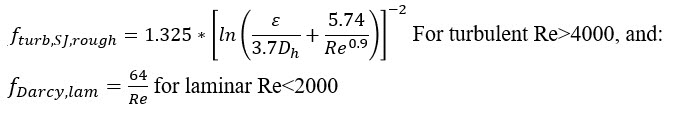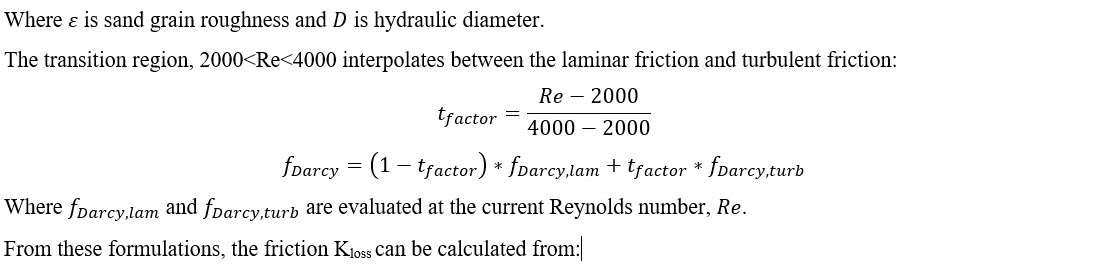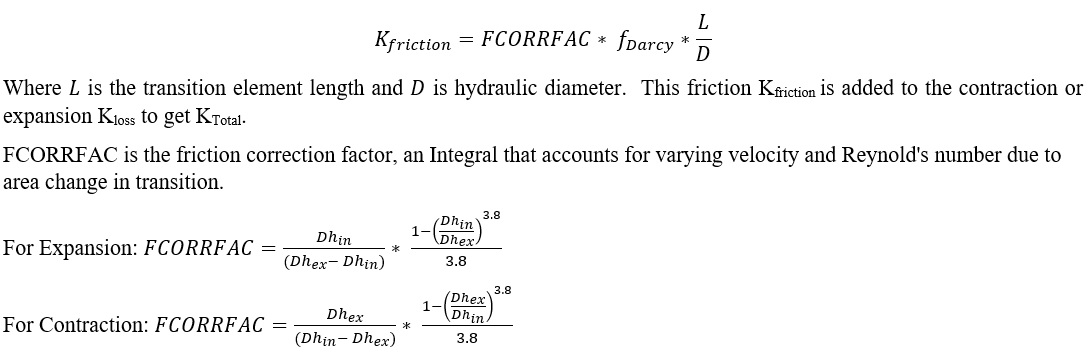Flow Rate Calculations

With the above in mind for how K (or Cp) is calculated for each of the transition element types, the momentum equation for each of the possible cases is outlined in the table 14 below. These equations can be used to derive an expression for mass flow rate,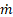.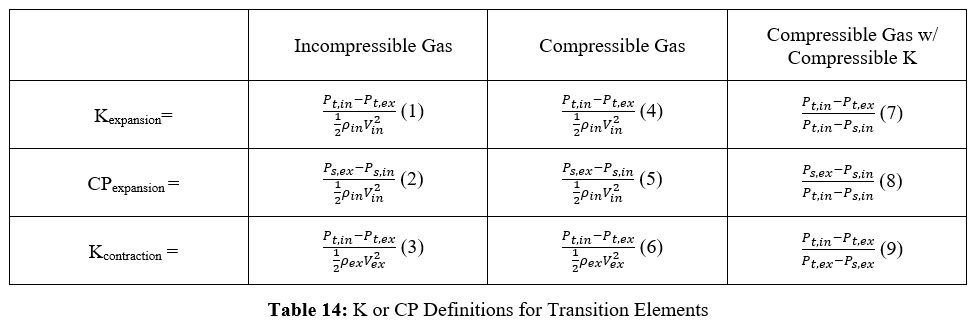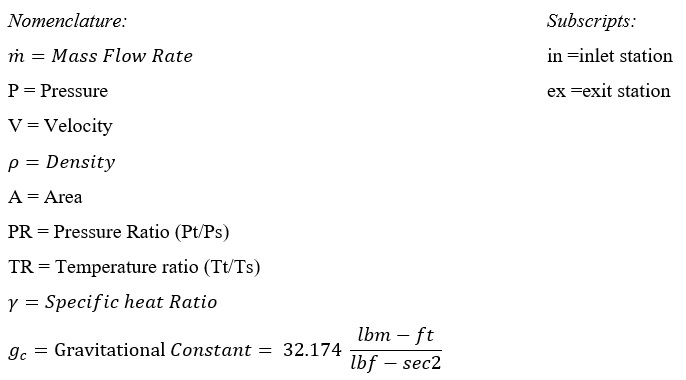Formulation offor (1) – K­expansion for an Incompressible Gas: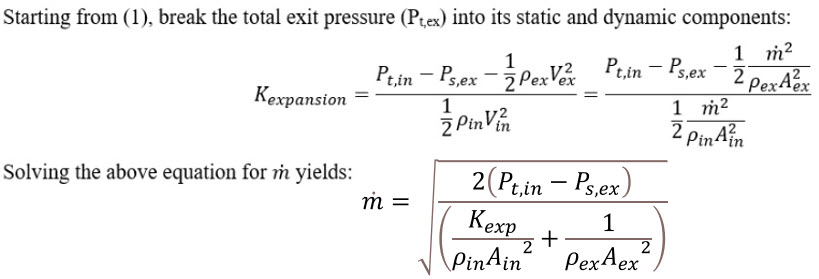Formulation offor (2) – CPexpansion for an Incompressible Gas: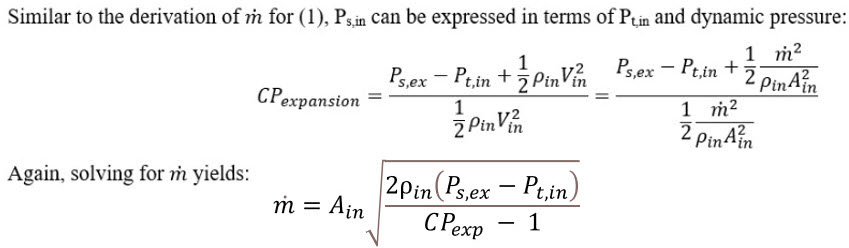Formulation offor (3) – Kcontraction for an Incompressible Gas: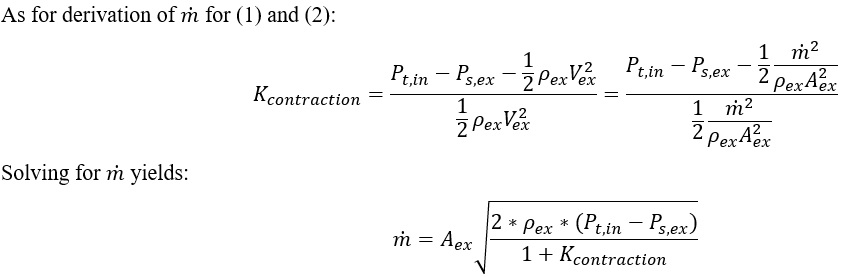Formulation offor (4) – Kexpansion for a Compressible Gas: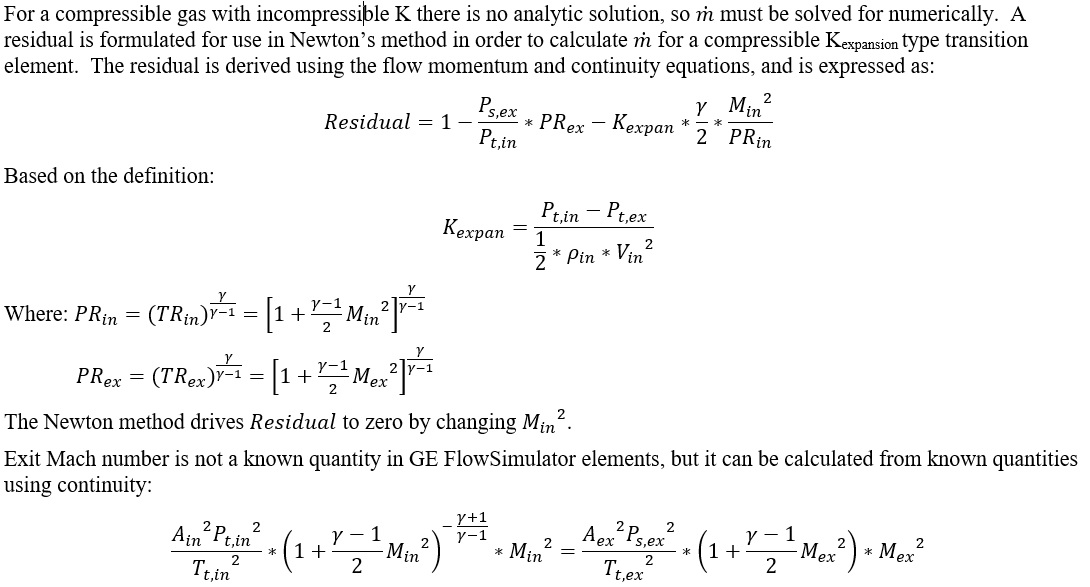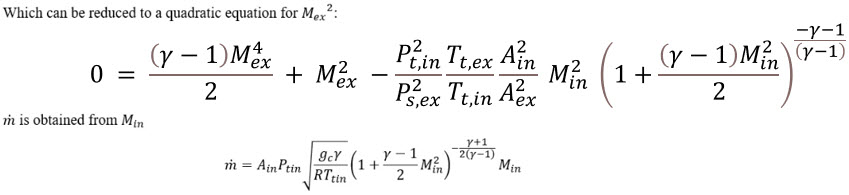Formulation offor (5) – CPexpansion for a Compressible Gas: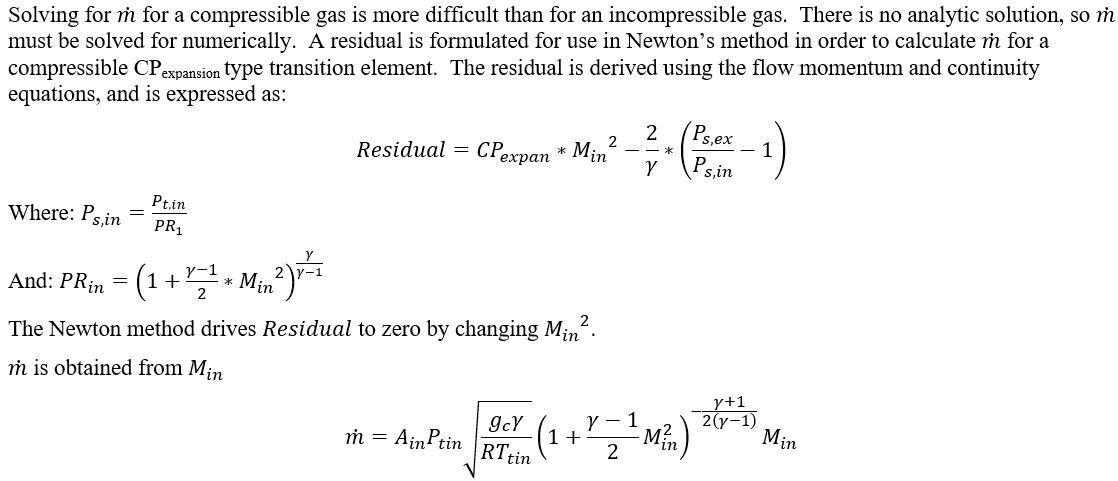Formulation offor (6) – Kcontraction for a Compressible Gas: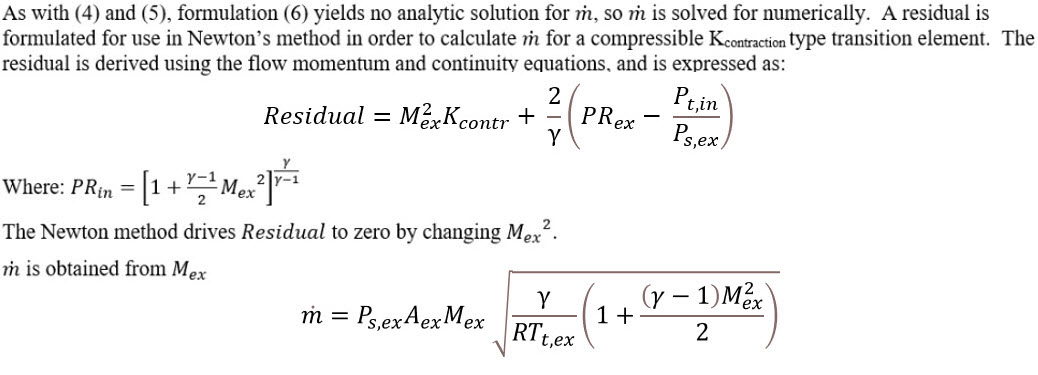Formulation offor (7) – Kexpansion for a Compressible Gas with Compressible K: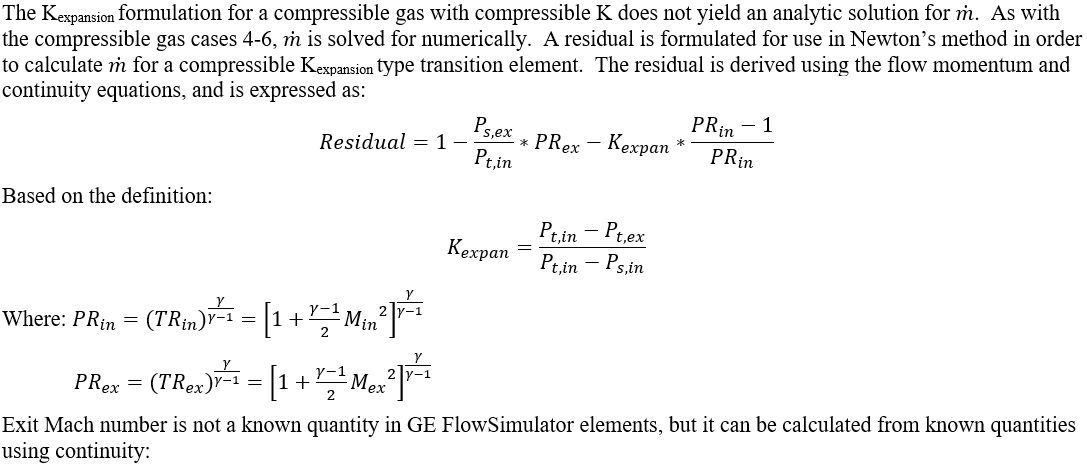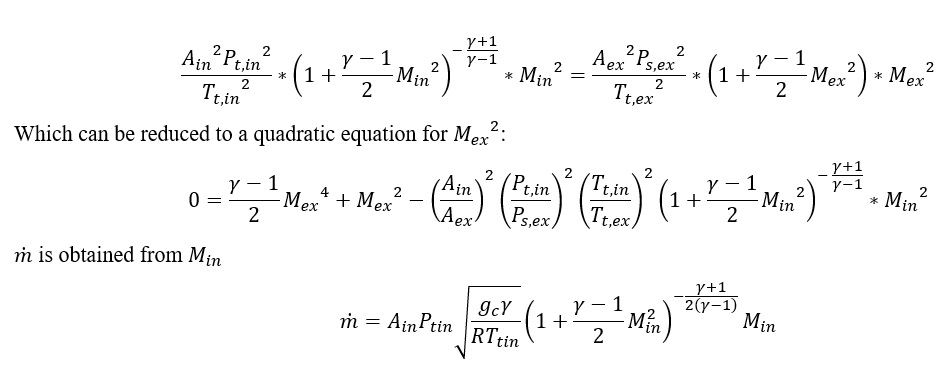Formulation offor (8) – CPexpansion for a Compressible Gas with Compressible CP: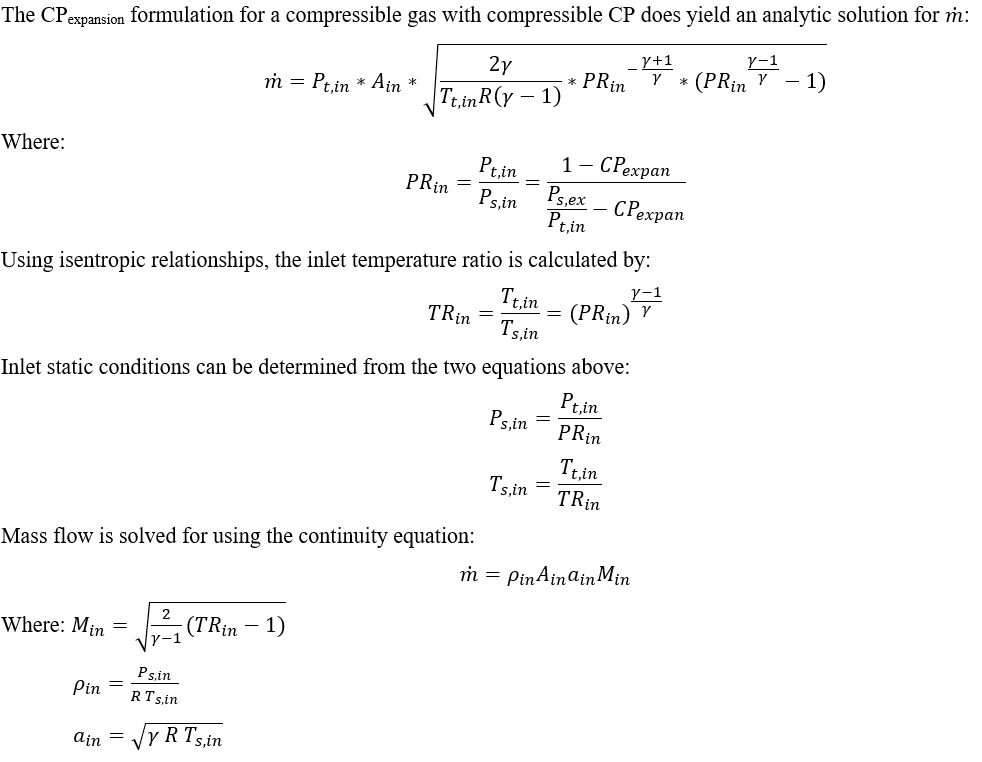Formulation offor (9) – Kcontraction for a Compressible Gas with Compressible K: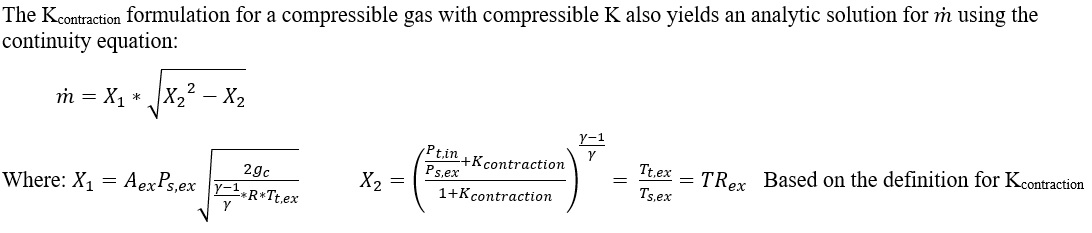For Additional Momentum loss, Portion of Upstream Dynamic Head loss, Exit K Loss refer Solver General theory section.

## Transition Element Outputs

Outputs in file with “res” extension. Output units controlled by user setting in “Output Control” panel.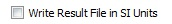Name Description Units ENG, SI
GEOMETRY: Expansion or contraction type that was finally used (which depends on flow direction). A list of possible geometries is as follows.

EXPANSION_W_FIXED_K

CONICAL_EXPANSION_BLEVINS

CONICAL_EXPANSION_MILLER

EXPANSION_W_FIXED_CP

EXPANSION_W_FIXED_EFFEC

ABRUPT_EXPANSION_SIMPLE

ABRUPT_EXPANSION_MILLER

CD_LOSS

CONTRACTION_W_FIXED_K

ABRUPT_CONTRACTION_SIMPLE

ABRUPT_CONTRACTION_POLYNOM

ABRUPT_CONTRACTION_MILLER1

ABRUPT_CONTRACTION_MILLER2

CONICAL_CONTRACTION_BLEVINS

CONICAL_CONTRACTION_MILLER

(None)
CROSS-SECTION: Shape of channel cross-section (CIRCULAR or ARBITRARY) (None)
FRICTION: How friction was calculated (OFF, SWAMEE, or FIXED) (None)
FLUID: Fluid compressibility option.

(INCOMPRESSIBLE, COMPRESSIBLE, or FULLY_COMPRESS)

Fully compressible means the fluid is compressible and the K-relationship is compressible too.

(None)
LENGTH Length of the transition channel inch, mm
INLET_AREA Area at Transition inlet inch2, m2
EXIT_AREA Area at Transition exit inch2, m2
DHIN Hydraulic Diameter at Transition inlet inch, mm
DHEX Hydraulic Diameter at Transition exit inch, mm
ROUGHNESS_TYPE: Type of roughness being used.

SAND_GRAIN

AVERAGE_ABSOLUTE

ROOT_MEAN_SQUARE

PEAK_TO_VALLEY

(None)
ROUGHNESS Roughness value (copy of input) inch, mm
EQUIV_SAND_GRAIN_ROUGHNESS Roughness value converted into a Sand-grain roughness so it can be used with the Colebrook-White relation inch, mm
FMULT Friction multiplier (copy of input, typically used in place of roughness) (unitless)
DARCY_FRIC Darcy friction factor at throat station (unitless)
FCORRFAC Friction loss correction factor that accounts for inlet and exit area being different. If Ain=Aex, then FCORRFAC=1. (unitless)
KFRIC Loss coefficient attributable to friction (unitless)
K_EXPAN Expansion K parameter (Total Pressure Loss Coefficient) for this expansion geometry (could be a user input, calculated value, or table lookup depending on the setup) (unitless)
CP_EXPAN Expansion Cp (Static Pressure Recovery Coefficient) for this expansion geometry (could be a user input, calculated value, or table lookup depending on the setup) (unitless)
EFFEC_EXPAN Diffuser Effectiveness for this expansion geometry (could be a user input, calculated value, or table lookup depending on the setup) (unitless)
K_EXPAN_TOTAL Combination of K_EXPAN and friction effects (unitless)
CP_TOT Combination of CP_EXPAN and friction effects (unitless)
EFFEC_TOT Combination of EFFEC_EXPAN and friction effects (unitless)
K_CONTRAC Contraction K loss for this contraction geometry (could be a user input, calculated value, or table lookup depending on the setup) (unitless)
K_CONTRAC_TOT Combination of K_CONTRAC and friction effects (unitless)

Fillet radius at the throat (just a copy of the input value)

Used only for the following sub-types:

ABRUPT_CONTRACTION_MILLER2

inch, mm
K_EXPAN_RESULT Back-calculated K. Should be the same as K_EXPAN_TOT (unitless)
CP_RESULT Back-calculated Cp. Should be the same as CP_TOT (unitless)
EFFEC_RESULT Back-calculated Effec. Should be the same as EFFEC_TOT (unitless)
K_CONTRAC_RESULT Back-calculated K. Should be the same as K_CONTRAC_TOT (unitless)
CD_RESULT Result calculated from actual mass flow rate divided by ideal mass flow rate. The ideal mass flow rate assumes either K=0, Cp=Cp_ideal, or Effec=1. (unitless)
FLUID_EXIT_THETA Exit Theta angle of fluid after going through K-exit losses (only shows if there are K-exit losses) deg
FLUID_EXIT_PHI Exit Phi angle of fluid after going through K-exit losses (only shows if there are K-exit losses) deg
PTS Driving pressure relative to the rotational reference frame (i.e. rotor) at the transition inlet. psia, MPa
PSIN

Static pressure relative to the rotational reference frame (i.e. rotor) at the transition inlet.

Limited by critical pressure ratio for supersonic flows when inlet area is smaller than exit area.

psia, MPa
PTEX Total pressure relative to the rotational reference frame (i.e. rotor) at the transition exit including supersonic effects. psia, MPa
PSEX

Static pressure relative to the rotational reference frame (i.e. rotor) at the transition exit.

Limited by critical pressure ratio for supersonic flows.

psia, MPa
PSEB Effective sink (static) pressure downstream of the transition. psia, MPa
TTS Total temperature of fluid relative to the rotational reference frame (i.e. rotor) at the transition inlet. deg F, deg K
TSIN Static temperature of fluid relative to the rotational reference frame (i.e. rotor) at the transition inlet. deg F, deg K
TTEX Total temperature of fluid relative to the rotational reference frame (i.e. rotor) at the transition exit. deg F, deg K
TSEX Static temperature of fluid relative to the rotational reference frame (i.e. rotor) at the transition exit. deg F, deg K

Positive values indicate heat added to the fluid; negative values indicate heat removed.

Btu/s, W
INVEL Velocity of fluid relative to the rotational reference frame (i.e. rotor) at the transition inlet. ft/s, m/s
INMN Mach number of fluid relative to the rotational reference frame (i.e. rotor) at the transition inlet. (unitless)
INREYN Reynolds number of fluid at the transition inlet. (unitless)
EXVEL Velocity of fluid relative to the rotational reference frame (i.e. rotor) at the transition exit. ft/s, m/s
EXMN Mach number of fluid relative to the rotational reference frame (i.e. rotor) at the transition exit. (unitless)
EXREYN Reynolds number of fluid at the transition exit. (unitless)

## References

1. Blevins, R. D., Applied Fluid Dynamics Handbook, Krieger Publications, 2003
2. Miller, D, Internal Flow Systems, Miller Innovations, 1990